### 6Parametric fonts and font macros

 (require sfont/parametric) package: sfont

The modules described above are useful for reading, modifying and inspecting fonts. However, to define new fonts sfont has macros tha should be easier to use.

#### 6.1Bezier paths

Instead of writing a bezier path point by point the macro ~ is provided, we will discuss how to use it with examples:

 syntax(~ path-element ...)

The simplest use is equivalent to specify a cubic bezier point by point

 > (~ (0 0) (55 0) (45 100) (100 100)) (list (vec 0 0) (vec 55 0) (vec 45 100) (vec 100 100))

A line is expressed with a double hyphen, -- (from now on the bezier paths will be printed with print-beziers):

 > (print-beziers (~ (0 0) -- (500 0) -- (500 500) -- (0 500) -- (0 0)))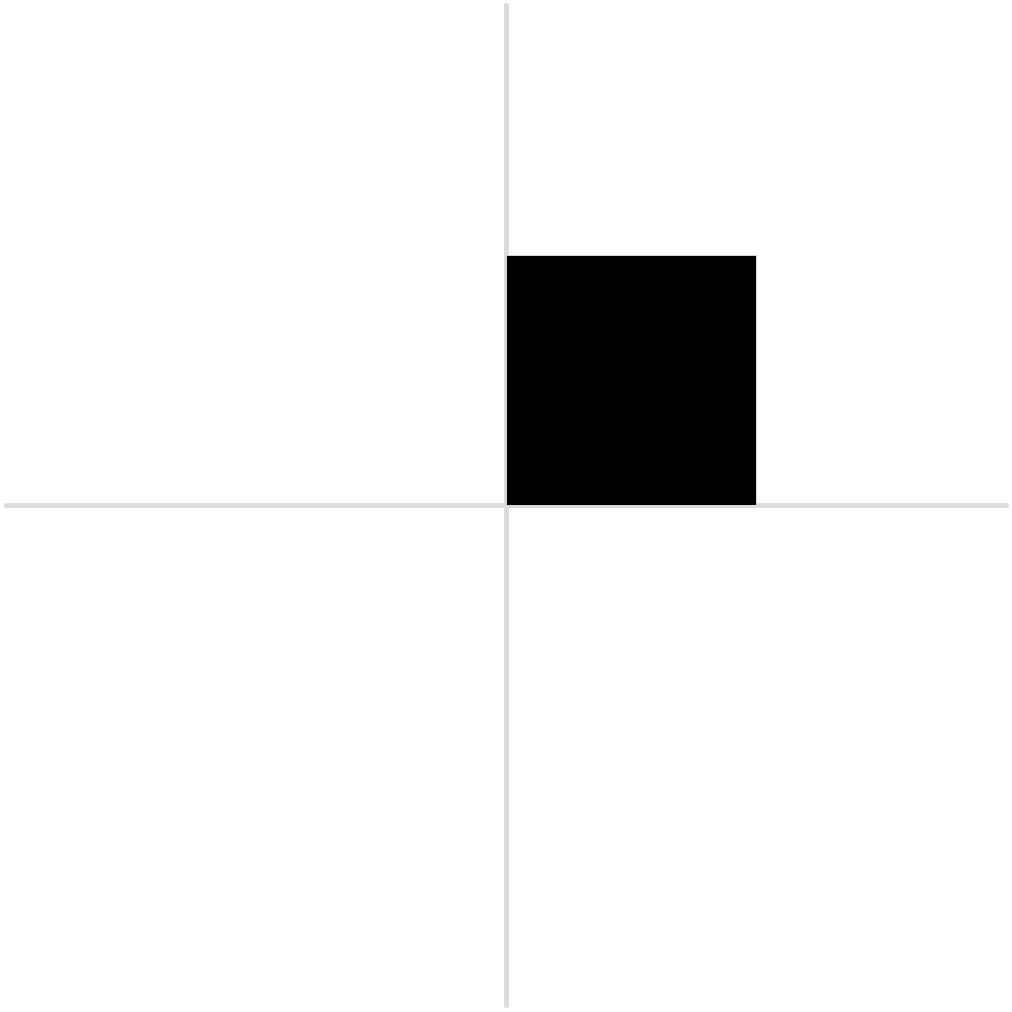To close the path, instead of repeating the firt point it is possible to use the cycle command:

 > (print-beziers (~ (0 0) -- (500 0) -- (500 500) -- (0 500) -- cycle))Coordinates can be relative to the last point:
 > (print-beziers (~ (0 0) -- (@ 500 0) -- (@ 0 500) -- (@ -500 0) -- cycle))Or using an angle and a distance
 > (print-beziers (~ (0 0) -- (@° 0 500) -- (@° (* pi 2/3) 500) -- cycle))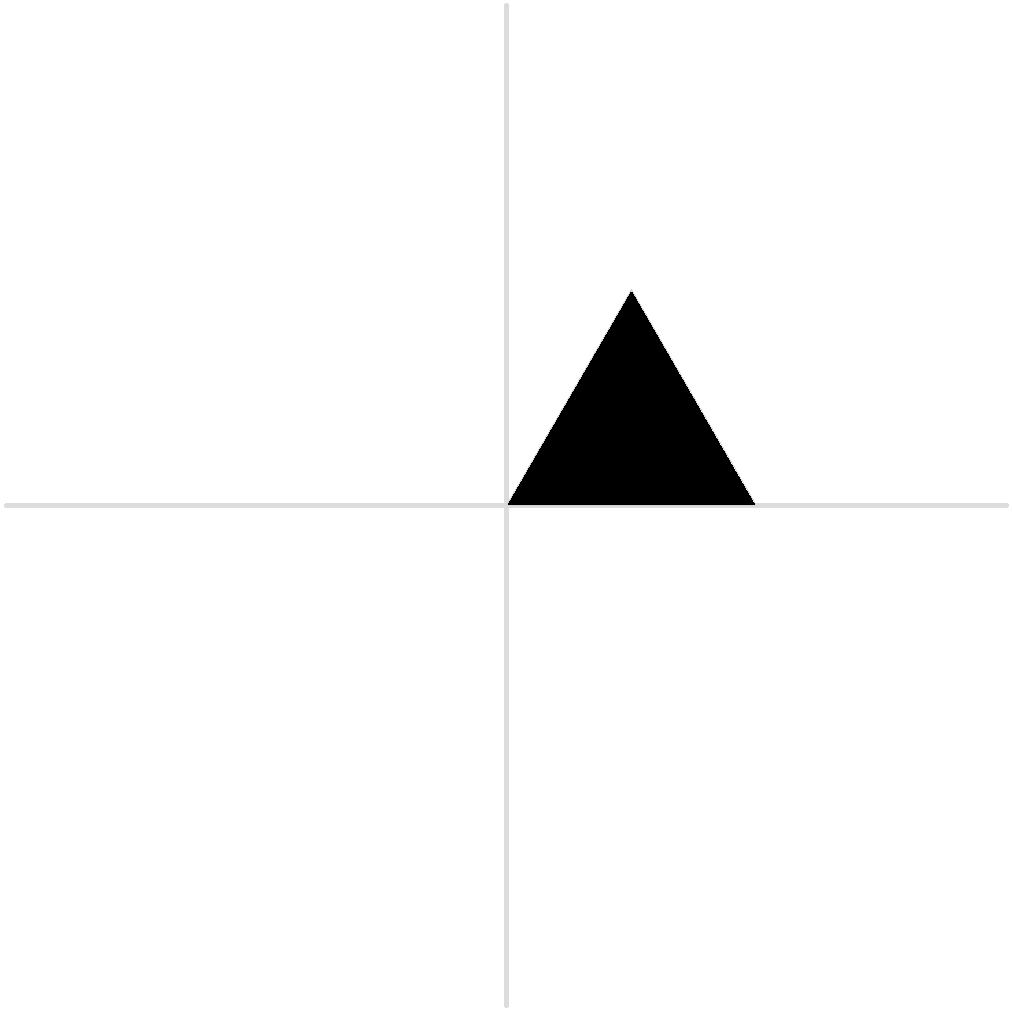Curve segments can be defined in an alternative way:
 > (print-beziers (~ (0 0) (500 0 0.55) (500 500) -- (0 500) -- cycle))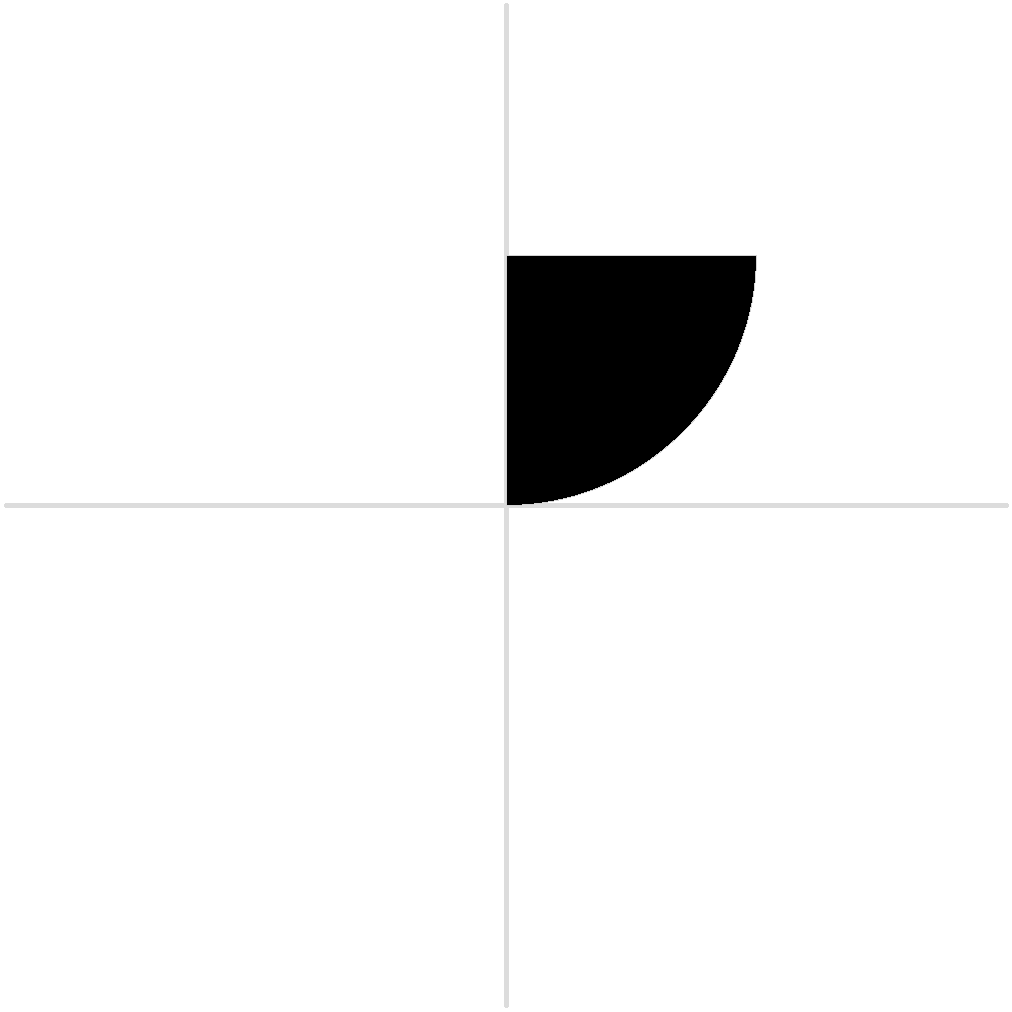where control points are obtained interpolating between (0 0) and (500 0) (with an interpolation factor 0.55) and between (500 500) and (500 0);

Finally we can insert another bezier inside ~ (the part inserted is joined to the rest with line segments).
> (define b1 (~ (100 0) -- (100 600)))
 > (print-beziers (~ (-100 0) (insert b1) cycle))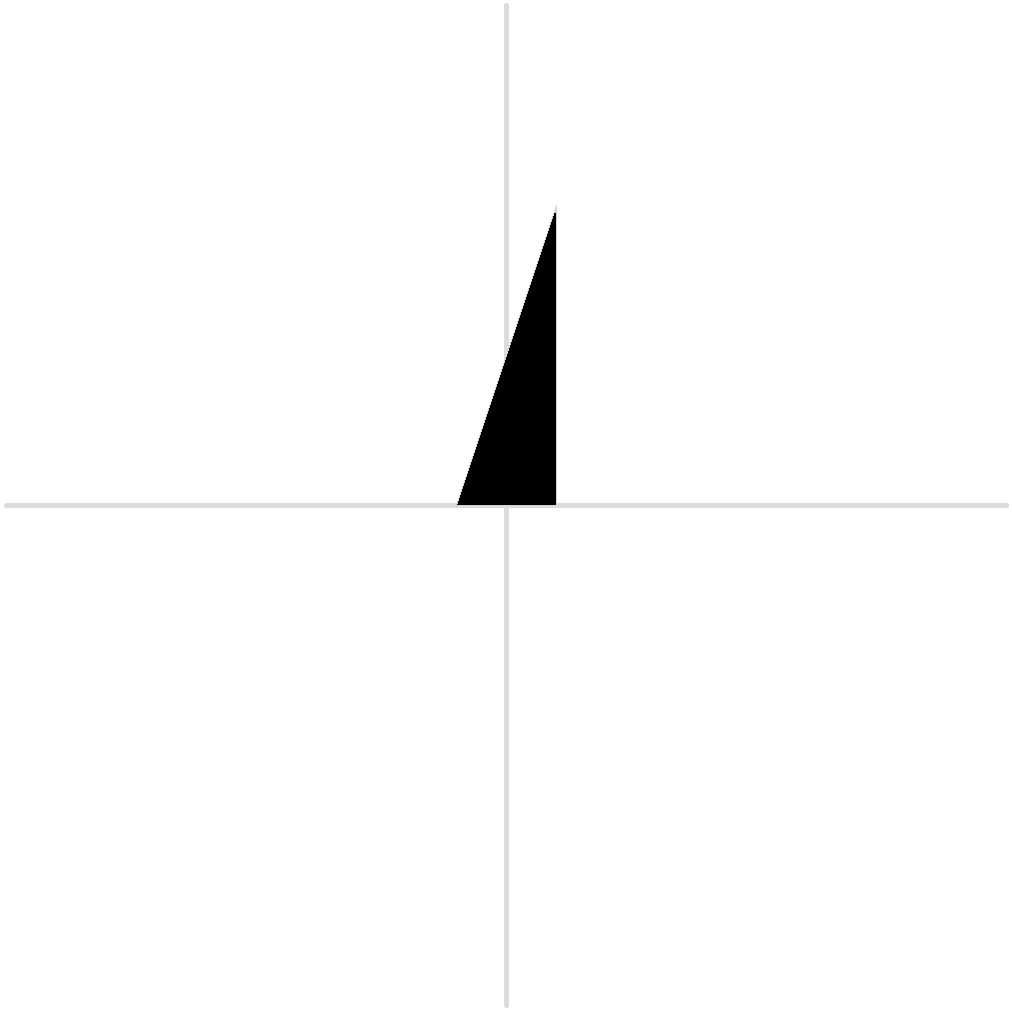#### 6.2Glyphs and Fonts

syntax

 (glyph. name maybe-locals [metrics left-form right-form] [contours contour ...] [components component ...])
 syntax(component. base) (component. base (sx sxy syx sy ox oy))

syntax

 (font. name [alignments alignment-form ...] maybe-variables [glyphs glyph-form ...])
 (font. (name (args default-value) ...) [alignments alignment-form ...] maybe-variables [glyphs glyph-form ...] maybe-spacing)

An example for glyphs:
 > (pict-convert (glyph. 'o [locals (weight 100) (width 400) (height 500)] [metrics 20 20] [contours (~ (0 0) -- (@ width 0) -- (@ 0 height) -- (@ (- width) 0) -- cycle) (~ (weight weight) -- (@ 0 (- height (* 2 weight))) -- (@ (- width (* 2 weight)) 0) -- (@ 0 (- (* 2 weight) height)) -- cycle)]))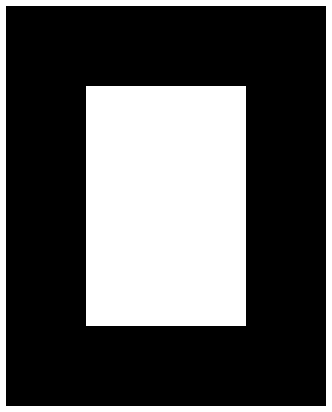Fonts can be defined in two ways: the first one creates a font, the second produces an anonymous procedure that can be called with the arguments defined and that produces a font.

 (define f (font. (squarefont (weight 100) (width 200)) ...))

f can be called with keyword arguments (f #:weight 120 #:width 210).

The body of the font can then use weight and width to create new fonts.

The creation of parametric fonts can then be made easier since the designer decide which ’parameter’ will be exposed, while other ’hidden’ variables can be defined in the maybe-variables part.

A complex example taken from the sfont-examples collection:

 (define sq (font. (squarefont  [x-height 500] [width 0.5] [weight 0.5]) (alignments [base 0 -10] [xh x-height 10] [desc* (/ (- x-height 1000) 2) 0 :font-descender] [asc* (- x-height (alg desc*)) 0 :font-ascender] [dsc (+ (alg desc*) 10) -10] [ascender (- (alg asc*) 10) 10]) (variables [gw (* 1000 width)] [v-stem (* x-height weight 0.333)] [h-stem (* v-stem 0.9)] [space (/ (- gw (* 2 v-stem)) 2)] [x1 space] ...) (glyphs (glyph. 'a (metrics space space) [contours a-cnt]) (glyph. 'b (metrics space (/--/ (+ gw space space))) [contours (rect x1 y1 v-stem (alg ascender)) (rect x1 y1 gw x-height) (reverse (rect (+ x1 v-stem) (+ y1 h-stem) (- gw (* 2 v-stem)) (- x-height (* 2 h-stem))))]) ...)))

Alignments are given in the form [name position overshoot-height], to get the values back the procedures alg, ovs, ovs-height, are defined:

 value

 procedure(alg a) → real? a : alignment/c
Produces the position of the alignment.

 procedure(ovs a) → real? a : alignment/c
Produces the position of the overshoot for the given alignment.

 procedure(ovs-height a) → real? a : alignment/c
Produces the height of the oveshoot for the given alignment.

#### 6.3Other functions and macros

 procedure(rect x y w h) → cubic-bezier/c x : real? y : real? w : real? h : real?
Produces a cubic bezier path of a rectangle. x, and y represent the lower left corner, w and h, the width and height.

 procedure(ellipse x y w h) → cubic-bezier/c x : real? y : real? w : real? h : real?
Produces a cubic bezier path of an ellipse. x, and y represent the lower left corner, w and h, the width and height.

 procedure(arc cx cy r a) → cubic-bezier/c cx : real? cy : real? r : real? a : real?
Produces a cubic bezier path of an open arc. cx, and cy represent the center, a and r, the angle and radius.

 procedure(remove~ pt rm ...) → (listof (and/c closed-bezier/c cubic-bezier/c)) pt : (and/c closed-bezier/c cubic-bezier/c) rm : (and/c closed-bezier/c cubic-bezier/c)
Remove the paths rm from pt.

 procedure(join~ pt pts ...) → (listof (and/c closed-bezier/c cubic-bezier/c)) pt : (and/c closed-bezier/c cubic-bezier/c) pts : (and/c closed-bezier/c cubic-bezier/c)
Add the paths pts to pt (remove overlaps).

 syntax(translate. o arg tx ty) (translate. o from (x y) arg tx ty)
 syntax(rotate. o arg angle) (rotate. o from (x y) arg angle)
 syntax(scale. o arg fx [fy fx]) (scale. o from (x y) arg fx [fy fx])
 syntax(skew-x. o arg angle) (skew-x. o from (x y) arg angle)
 syntax(skew-y. o arg angle) (skew-y. o from (x y) arg angle)
 syntax(reflect-x. o arg) (reflect-x. o from (x y))
 syntax(reflect-y. o arg) (reflect-y. o from (x y) arg)
Like the corresponding transformations, but can be used with bezier paths and with the from command that specifies the center of trasformation.